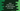# Java program to find the sum of all odd numbers in an array## Java program to find the sum of all odd numbers in an array:

This post will show you how to find the total sum of all odd numbers in an array of numbers in Java. This program will take the numbers as input from the user, insert all in an array and print out the sum.

Below is the complete algorithm that we will use:

### Algorithm:

• Read the size of the array from the user.
• Create one array with size equal to the user input value.
• Read the numbers from the user and insert them in the array.
• Create one variable to store the count and initialize it as 0.
• Run one for loop and iterate through the array. For each element, check if it is odd or not. If it is odd, then add it to the sum.
• At the end of the program, print the value of sum.

We can use a for loop or while loop to solve it. I will show you three different ways to count the odd numbers in a user-given array.

### Method 1: Find all odd numbers in an array using for loop:

Below is the complete program:

``````import java.util.Scanner;

public class Main {

public static void main(String[] args) {
Scanner sc = new Scanner(System.in);

System.out.println("Enter the size of the array : ");
int size = sc.nextInt();

int[] intArr = new int[size];

System.out.println("Enter the values : ");
for (int i = 0; i < size; i++) {
intArr[i] = sc.nextInt();
}

int sum = 0;
for (int i = 0; i < size; i++) {
if (intArr[i] % 2 != 0) {
sum += intArr[i];
}
}

System.out.println("Sum of all odd numbers in the array is : " + sum);
}
}``````

Here,

• It reads the size of the array from the user and stores it in the variable size
• It creates an array of integers intArr to hold the user input values. Using a for loop, it reads the user input numbers and put them in intArr.
• sum variable is initialized with 0. This variable is used to store the sum of all odd numbers in the array.
• Using another for loop, it is iterating through the integer array one by one. For each number, it is checking if it is odd or not. If it is odd, then it adds that value to the sum variable.
• At the end, it prints the value of sum, i.e. the sum of all odd numbers in the array.

It will print output as like below:

``````Enter the size of the array :
5
Enter the values :
1 2 3 4 5
Sum of all odd numbers in the array is : 9``````

### Method 2: Find the sum of odd numbers in an array using a while loop:

We can also use a while loop to find the sum of all odd numbers in an array. Below is the complete program:

``````import java.util.Scanner;

public class Main {

public static void main(String[] args) {
Scanner sc = new Scanner(System.in);

System.out.println("Enter the size of the array : ");
int size = sc.nextInt();

int[] intArr = new int[size];

System.out.println("Enter the values : ");
for (int i = 0; i < size; i++) {
intArr[i] = sc.nextInt();
}

int sum = 0;
int i = 0;
while (i < size) {
if (intArr[i] % 2 != 0) {
sum += intArr[i];
}
i++;
}

System.out.println("Sum of all odd numbers in the array is : " + sum);
}
}``````

It will give output similar to the above.

### Method 3: Find the sum of odd numbers in an array using a different method:

We can put the part to find out the sum in a different method. That will make it easy to call this method from different parts of the application:

``````import java.util.Scanner;

public class Main {

public static int findOddSum(int[] arr, int size){
int sum = 0;
int i = 0;
while (i < size) {
if (arr[i] % 2 != 0) {
sum += arr[i];
}
i++;
}
return sum;
}

public static void main(String[] args) {
Scanner sc = new Scanner(System.in);

System.out.println("Enter the size of the array : ");
int size = sc.nextInt();

int[] intArr = new int[size];

System.out.println("Enter the values : ");
for (int i = 0; i < size; i++) {
intArr[i] = sc.nextInt();
}

System.out.println("Sum of all odd numbers in the array is : " + findOddSum(intArr, size));
}
}``````

It prints similar output.